output.to from Sideway
Draft for Information Only

# Content

```Second Moment of Mass  Moment of Inertia of a Thin Plate  Moment of Inertia of Thin Circular Plate  Moment of Inertia of Thin Rectangular Plate```

# Second Moment of Mass

Similarly, the second moment of mass about an axis is equal to the summation of the products of the square of the distance between the elemental mass and the reference axis, and the elemental mass over an area. As the second moment of mass is usually refered to the rotation, the distance between the elemental mass and the rotating axis is denoted by r. Imply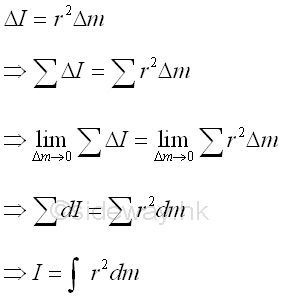## Moment of Inertia of a Thin Plate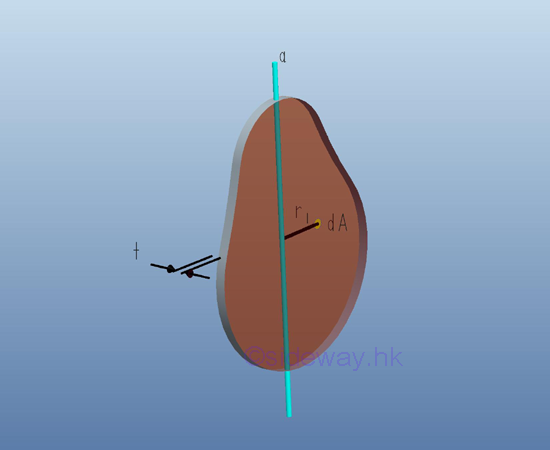Consider a thin plate of area A with uniform thickness t and homogenouse material density ρ. Both the thickness and the material density are constant over the area. Since the thickness t is much smaller than the plate dimension, the mass and the elemental mass of the thin plate can be expressed in terms of the thickness and the material density, if the reference axis lies in the middle plane of the plate, the mass moment of inertia of the thin plate with respect to an axis can be expressed as the elemental area on the middle plane with the distance r as the radius between the elemental area and the axis. ImplyTherefore for a uniform homogenous thin plate,  the mass moment of inertia about axis a can be expressed in terms of area moment of inertia about axis a. Imply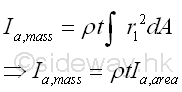For the mass moment of inertia about axis b, which is perpendicular to axis a.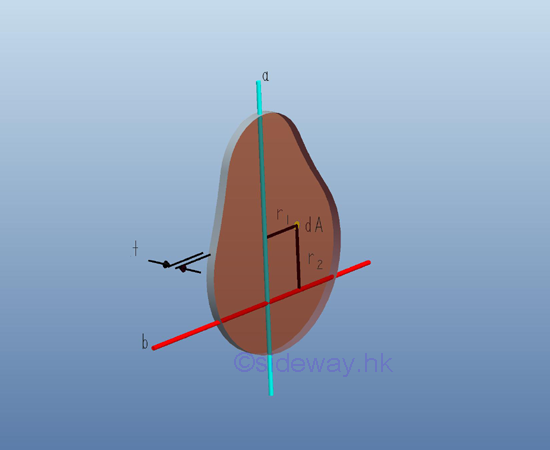Similarly, the mass moment of inertia about axis b, which is perpendicular to axis a can be expressed in terms of the area moment of inertia about b as in the rectangular moments of inertia. ImplyAnd for the mass moment of inertia about axis c, which is perpendicular to the plate and pass through the intersection of axes a and b.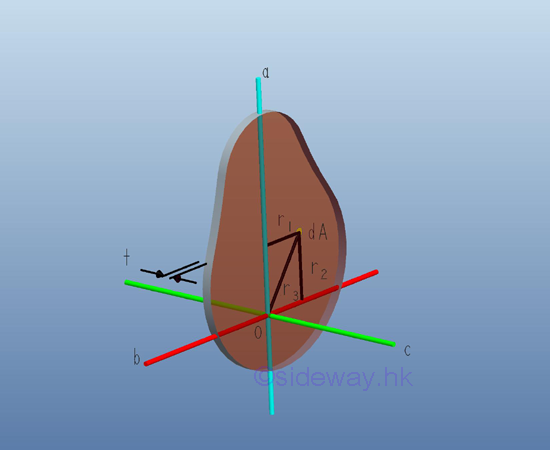Similarly, the mass moment of inertia about axis c, which is perpendicular to the plate and pass through the intersection of axes a and b can be expressed in term of the area polar moment of inertia about pole O in magnitude but the mass moment of inertia is concerning about the inertia of rotation about the axis c. Imply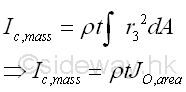Therefore, from the relation between the rectangular area moments of inertia and the polar area moment of inertia, the relationship for the rectangular mass moments of inertia of a unform homogenous thin plate is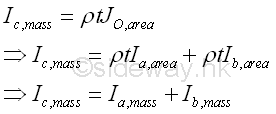## Moment of Inertia of Thin Circular Plate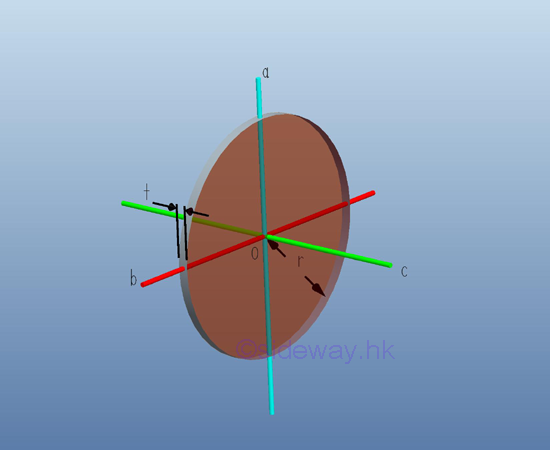For a thin uniform homogenous circular plate, the mass moment of inertia about the rectangular coordinate axes, a and b, passing through the centre of gravity of the circular plate can be obtained from the area moment of inertia. ImplySimilarly, the mass moment of inertia about axis c perpendicular to the rectangular coordinate axes a and b can also be obtained from the relation between the polar area moment of inertia and the rectangular area moment of inertia. Imply## Moment of Inertia of Thin Rectangular Plate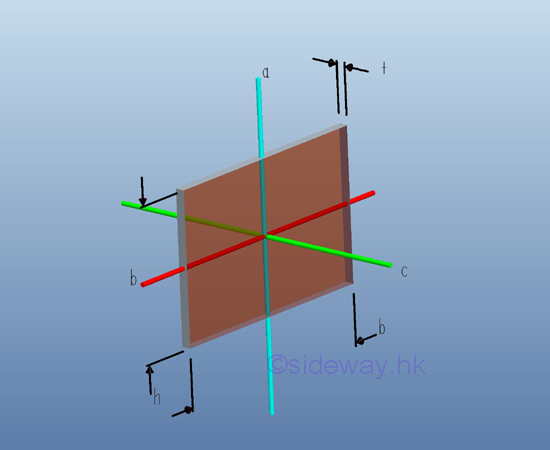For a thin uniform homogenous rectangular plate, the mass moment of inertia about the rectangular coordinate axes, a and b, passing through the centre of gravity of the circular plate can be obtained from the area moment of inertia. Imply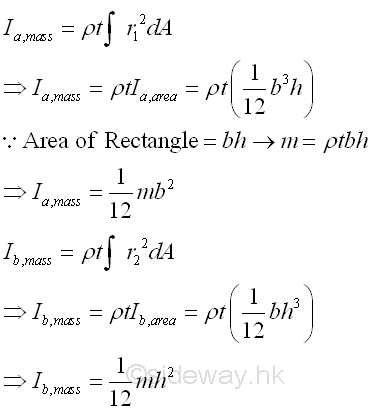Similarly, the mass moment of inertia about axis c perpendicular to the rectangular coordinate axes a and b can also be obtained from the relation between the polar area moment of inertia and the rectangular area moment of inertia. Imply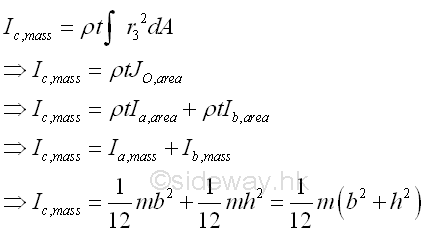ID: 121100002 Last Updated: 10/16/2012 Revision: 0 Ref:References

1. I.C. Jong; B.G. rogers, 1991, Engineering Mechanics: Statics and Dynamics
2. F.P. Beer; E.R. Johnston,Jr.; E.R. Eisenberg, 2004, Vector Mechanics for Engineers: StaticsHome 5

Management

HBR 3

Information

Recreation

Culture

Chinese 1097

English 339

Computer

Hardware 224

Software

Application 213

Latex 52

Manim 204

KB 1

Numeric 19

Programming

Web 289

Unicode 504

HTML 66

CSS 65

SVG 46

ASP.NET 270

OS 429

Python 72

Knowledge

Mathematics

Algebra 84

Geometry 33

Calculus 67

Engineering

Mechanical

Rigid Bodies

Statics 92

Dynamics 37

Control

Natural Sciences

Electric 27